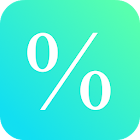Percent Calculator

All Android applications categories

All Android games categories# Percent Calculator

by: 1.3K 8.4

8.4 Users
rating

## Screenshots

Description

This free math calculator lets you calculate percentages from a real number.

Best mathematical tool for school and college! If you are a student, it will helps you to learn percent calculations with numbers.

Note: In mathematics, a percentage is a number or ratio expressed as a fraction of 100. It is used to calculate increase and decrease, concentration, interest and so on.

Tags: como calcular el 10 por ciento de un numero , パーセントの計算

## Users review

from 1.341 reviews

"Great"

8.4